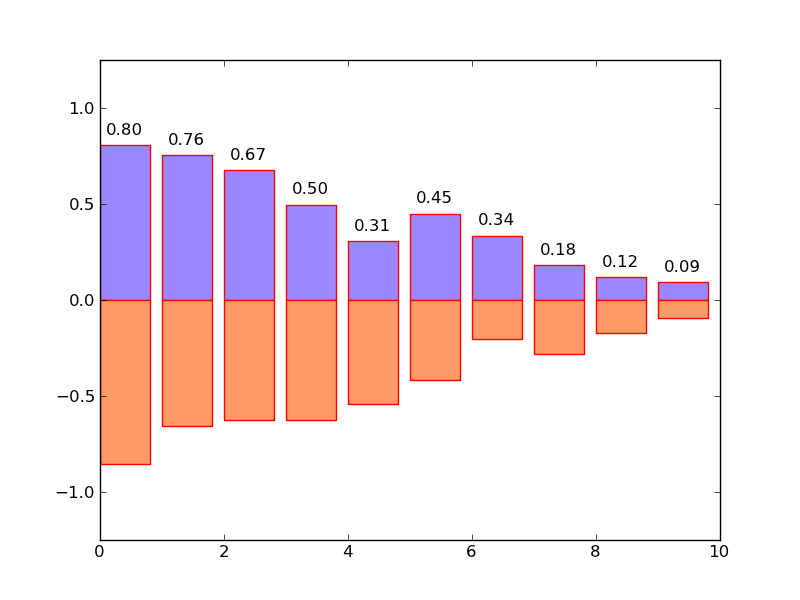python数据分析之（7）简单绘图pylab

pylab 是 matplotlib 面向对象绘图库的一个接口，它的语法和 Matlab 十分相近，主要的绘图命令和 Matlab 对应的命令有相似的参数。简单的实例：

from pylab import *
X = np.linspace(-np.pi, np.pi, 256,endpoint=True)
C,S = np.cos(X), np.sin(X)
plot(X,C)
plot(X,S)
show()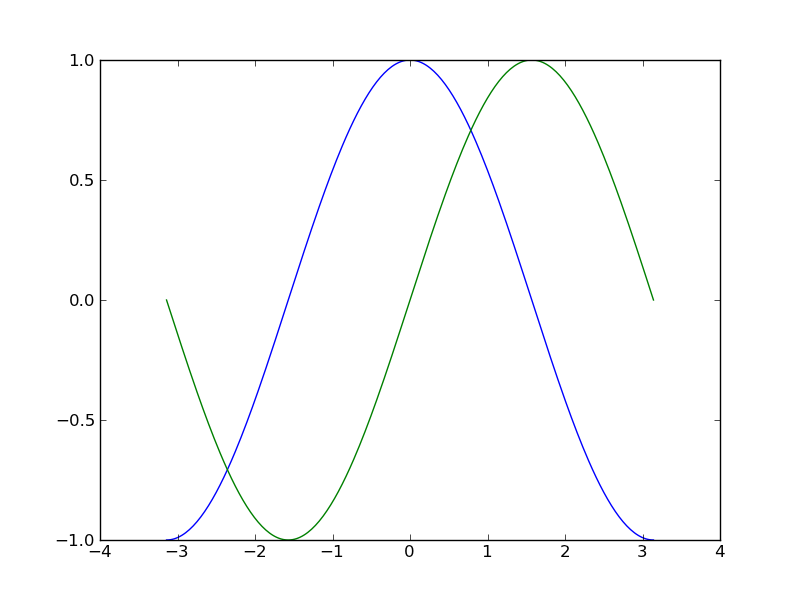X 是一个 numpy 数组，包含了从 −π到 +π等间隔的 256 个值。C 和 S 则分别是这 256 个值对应的余弦和正弦函数值组成的numpy 数组。另外，Matplotlib 的默认配置都允许用户自定义。你可以调整大多数的默认配置：图片大小和分辨率（dpi）、线宽、颜色、风格、坐标轴、坐标轴以及网格的属性、文字与字体属性等。

from pylab import *

# 创建一个 8 * 6 点的图，设置分辨率为 80
figure(figsize=(8,6), dpi=80)

# 创建一个新的 1 * 1 的子图，接下来的图样绘制在其中的第 1 块（唯一的一块）
subplot(1,1,1)

X = np.linspace(-np.pi, np.pi, 256,endpoint=True)
C,S = np.cos(X), np.sin(X)

# 绘制余弦曲线，使用蓝色的、连续的、宽度为 1 （像素）的线条
plot(X, C, color="blue", linewidth=1.0, linestyle="-")

# 绘制正弦曲线，使用红色的、连续的、宽度为 1 （像素）的线条
plot(X, S, color="red", linewidth=1.0, linestyle="-")

# 设置横轴的上下限
xlim(-4.0,4.0)
# 设置横轴记号
xticks(np.linspace(-4,4,9,endpoint=True))

# 设置纵轴的上下限
ylim(-1.0,1.0)
# 设置纵轴记号
yticks(np.linspace(-1,1,5,endpoint=True))

# 在屏幕上显示
show()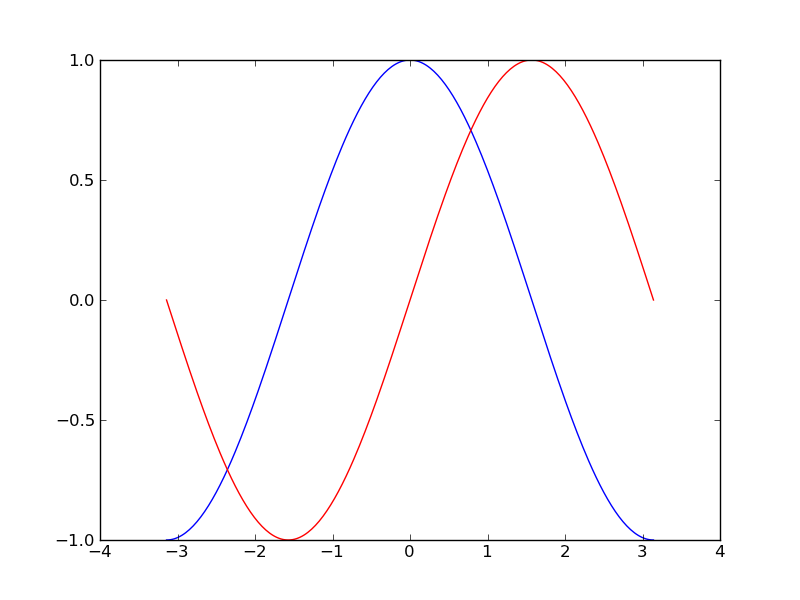from pylab import *

# 创建一个 8 * 6 点的图，设置分辨率为 80
figure(figsize=(8,6), dpi=80)

# 创建一个新的 1 * 1 的子图，接下来的图样绘制在其中的第 1 块（唯一的一块）
subplot(1,1,1)

X = np.linspace(-np.pi, np.pi, 256,endpoint=True)
C,S = np.cos(X), np.sin(X)

# 绘制曲线，添加图例
plot(X, C, color="blue", linewidth=1.0, linestyle="-", label="cos")
plot(X, S, color="red",  linewidth=1.5, linestyle="-", label="sin")
legend(loc='upper left')

# 设置横轴的上下限
xlim(-4.0,4.0)
# 设置横轴记号
xticks(np.linspace(-4,4,9,endpoint=True))

# 设置纵轴的上下限
ylim(-1.0,1.0)
# 设置纵轴记号
yticks(np.linspace(-1,1,5,endpoint=True))

# 在屏幕上显示
show()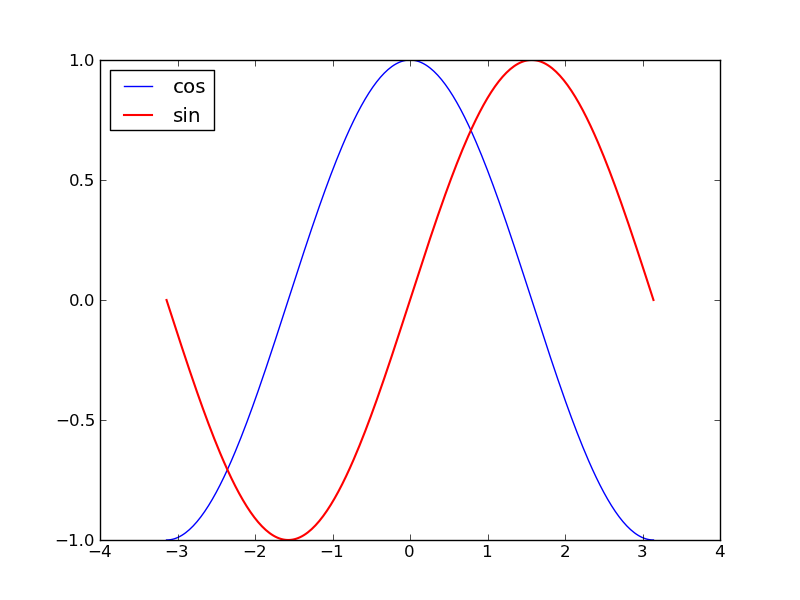Matplotlib 中的「图像」指的是用户界面看到的整个窗口内容。在图像里面有所谓「子图」。子图的位置是由坐标网格确定的，而「坐标轴」却不受此限制，可以放在图像的任意位置。我们已经隐式地使用过图像和子图：当我们调用 plot 函数的时候，matplotlib 调用 gca() 函数以及 gcf() 函数来获取当前的坐标轴和图像；如果无法获取图像，则会调用 figure() 函数来创建一个——严格地说，是用 subplot(1,1,1) 创建一个只有一个子图的图像。

from pylab import *

# 创建一个 8 * 6 点的图，设置分辨率为 80
figure(figsize=(8,6), dpi=80)

# 创建一个新的 2 * 1 的子图，接下来的图样绘制在其中的第 1 块
subplot(2,1,1)

X = np.linspace(-np.pi, np.pi, 256,endpoint=True)
C,S = np.cos(X), np.sin(X)

# 绘制曲线，添加图例
plot(X, C, color="blue", linewidth=1.0, linestyle="-", label="cos")
legend(loc='upper left')
# 创建一个新的 2 * 1 的子图，接下来的图样绘制在其中的第 2 块
subplot(2,1,2)
plot(X, S, color="red",  linewidth=1.5, linestyle="-", label="sin")
legend(loc='upper left')

# 设置横轴的上下限
xlim(-4.0,4.0)
# 设置横轴记号
xticks(np.linspace(-4,4,9,endpoint=True))

# 设置纵轴的上下限
ylim(-1.0,1.0)
# 设置纵轴记号
yticks(np.linspace(-1,1,5,endpoint=True))

# 在屏幕上显示
show()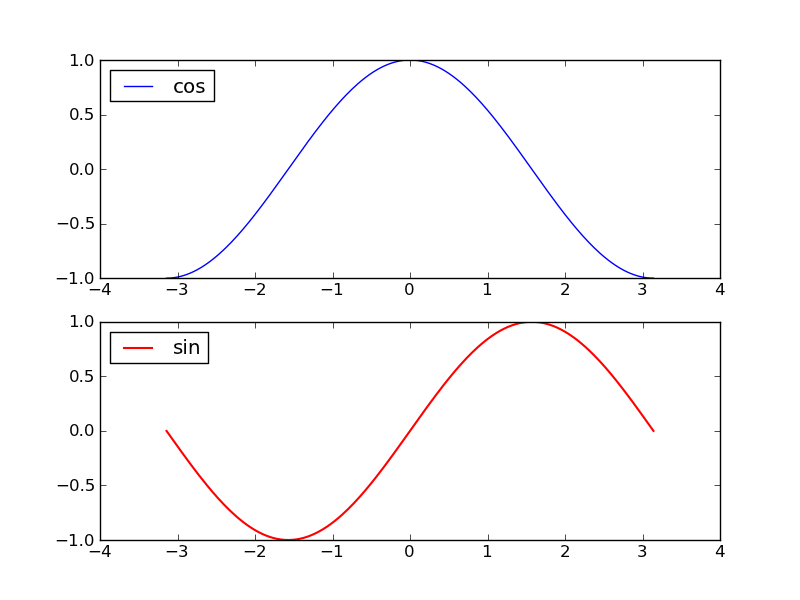from pylab import *

n = 1000
X = np.random.normal(0,1,n)
Y = np.random.normal(0,1,n)
#散点图
scatter(X,Y)
show()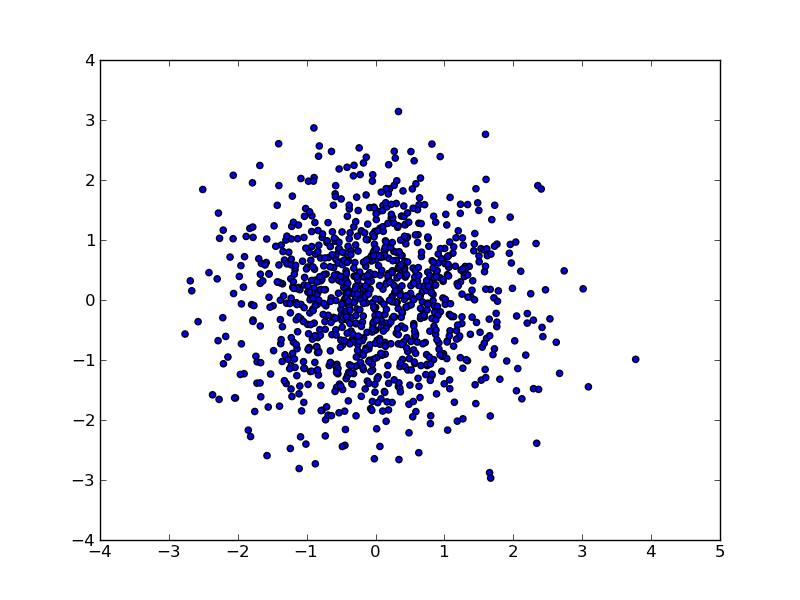from pylab import *

n = 10
X = np.arange(n)
Y1 = (1-X/float(n)) * np.random.uniform(0.5,1.0,n)
Y2 = (1-X/float(n)) * np.random.uniform(0.5,1.0,n)
#柱状图
bar(X, +Y1, facecolor='#9988ff', edgecolor='red')
bar(X, -Y2, facecolor='#ff9966', edgecolor='red')

for x,y in zip(X,Y1):
text(x+0.4, y+0.05, '%.2f' % y, ha='center', va= 'bottom')

ylim(-1.25,+1.25)
show()